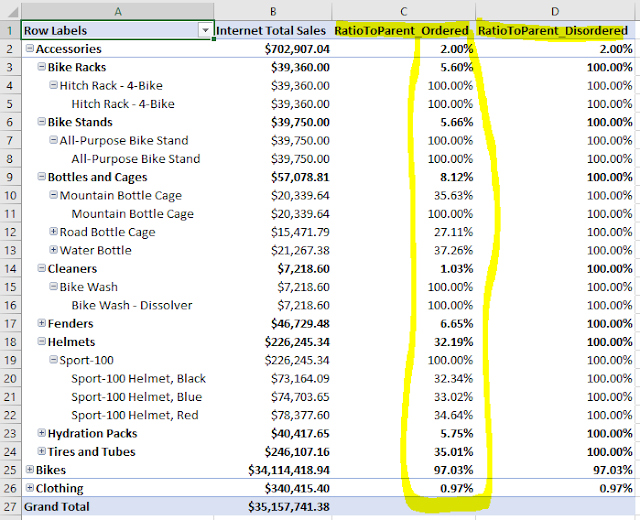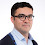### DAX calculations with hierarchies: Set the order straight.

[2017-Apr-30] If you have ever tried to create calculated measures in SSAS Tabular/ Power BI models where different levels of your model hierarchy had to be considered then you'd understand how DAX language doesn't have a straight way to identify parent & child levels in order to compute necessary metric results. There is a good article http://www.sqlbi.com/articles/clever-hierarchy-handling-in-dax/ by Alberto Ferrari where he explains a workaround approach to handle hierarchies in DAX.

I made one step further, based on my recent client project experience, and created a new example with one extra level for the Product dimension using Adventure Works Internet Sales tabular database. Here is the code to calculate Sales Ratio to a Parent level for all levels (Category, Sub Category, Model and Product Name)

```RatioToParent:=
IF (
ISFILTERED ( Product[HCategory] ),
SUM ( 'Internet Sales'[Sales Amount] )
/ CALCULATE ( SUM ( 'Internet Sales'[Sales Amount] ), ALL ( Product[HCategory] ) ),
IF (
ISFILTERED ( Product[HSubcategory] ),
SUM ( 'Internet Sales'[Sales Amount] )
/ CALCULATE ( SUM ( 'Internet Sales'[Sales Amount] ), ALL ( Product[HSubcategory] ) ),
IF (
ISFILTERED ( Product[HModel] ),
SUM ( 'Internet Sales'[Sales Amount] )
/ CALCULATE (
SUM ( 'Internet Sales'[Sales Amount] ),
ALL ( Product[HModel] )
),
IF (
ISFILTERED ( Product[HProduct] ),
SUM ( 'Internet Sales'[Sales Amount] )
/ CALCULATE ( SUM ( 'Internet Sales'[Sales Amount] ), ALL ( Product[HProduct] ) )
)
)
)
)
```

However, when I looked at the results for this new RatioToParent measure using Excel, I saw some weird values: only the to Category level showed me correct calculations and all other Product hierarchy levels had 100%.Then I changed the order of levels in my DAX calculated measure from (Category > Sub Category > Model > Product Name) to (Product Name >  Model > Sub Category Category):

```RatioToParent_Ordered:=
IF (
ISFILTERED ( Product[HProduct] ),
SUM ( 'Internet Sales'[Sales Amount] )
/ CALCULATE ( SUM ( 'Internet Sales'[Sales Amount] ), ALL ( Product[HProduct] ) ),
IF (
ISFILTERED ( Product[HModel] ),
SUM ( 'Internet Sales'[Sales Amount] )
/ CALCULATE ( SUM ( 'Internet Sales'[Sales Amount] ), ALL ( Product[HModel] ) ),
IF (
ISFILTERED ( Product[HSubcategory] ),
SUM ( 'Internet Sales'[Sales Amount] )
/ CALCULATE (
SUM ( 'Internet Sales'[Sales Amount] ),
ALL ( Product[HSubcategory] )
),
IF (
ISFILTERED ( Product[HCategory] ),
SUM ( 'Internet Sales'[Sales Amount] )
/ CALCULATE ( SUM ( 'Internet Sales'[Sales Amount] ), ALL ( Product[HCategory] ) )
)
)
)
)
```

and that made the whole difference and resolved the issue of miscalculated results:So my learning lesson in this exercise was that order of hierarchical levels in DAX calculations is very important. Otherwise, calculations can only be correctly fulfilled for the top parent level of your tabular model hierarchy (please see the first DAX calculations). Lesson learned, mistakes not to be repeated!

1.This makes sense, right. Once an if statement evaluates to TRUE the resulting expression is evaluated and the loop terminates. So if higher levels of your hierarchy are included in the pivot the first if statement will always evaluate to TRUE and the loop will never make it pass the first if statement.

2.Yes, I learned it the hard way of testing the data and then switching the order within my real project DAX calculation to begin from the leaf level to the top of my hiearchy.

3.Hi Rayis,

I onboarded PBI and DAX only recently, after working for 2 years with Qlik stack. What struck me with DAX is that if you change the structure of your pivot table, your DAX formula may break.

Is this the case also with this hierarchical formula you created? Imagine if we need to reshuffle the dimensions in the hierarchy, e.g., in a hypothetical example, put first Country, then Product Group, then Subsidiary, and then change their order to see different perspectives - will we need to adjust the sequence in the formula to get correct ratio to parent?

I am just asking this because I struggle a bit to grasp the DAX to a degree when I would intuitively understand what works and what not.

4.I agree with you Wylde, if you build your pivot table around separated hierarchical level, then yes, it's more fragile. The use of the solid hierarchy will give you more stability, but again all the DAX calculations has to be in sync with existing hierarchies' levels. I understand that DAX is not perfect yet...